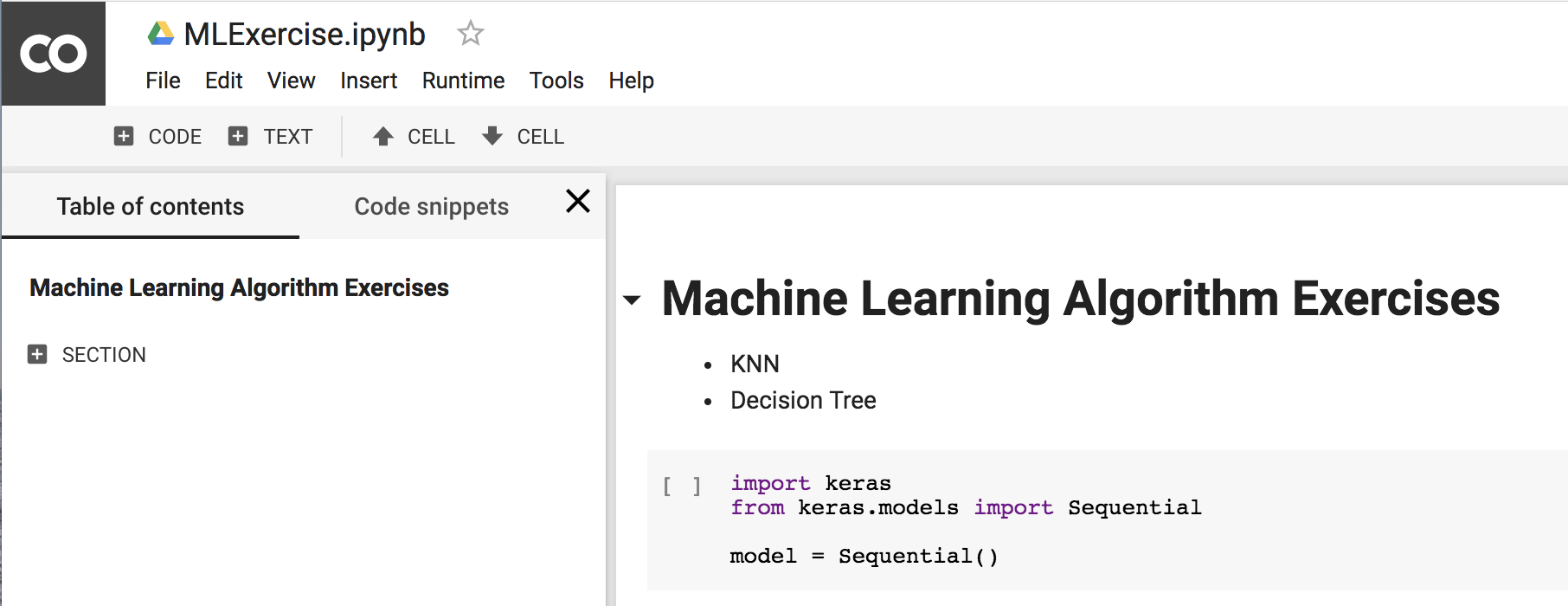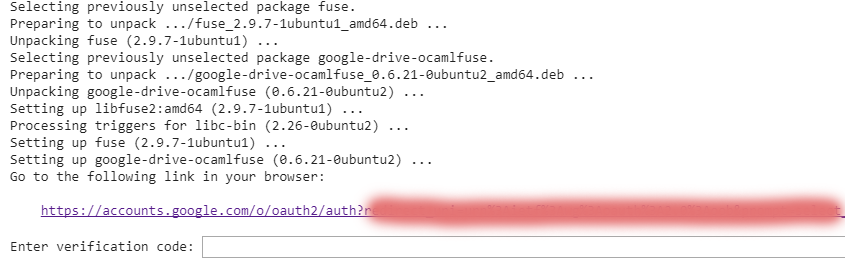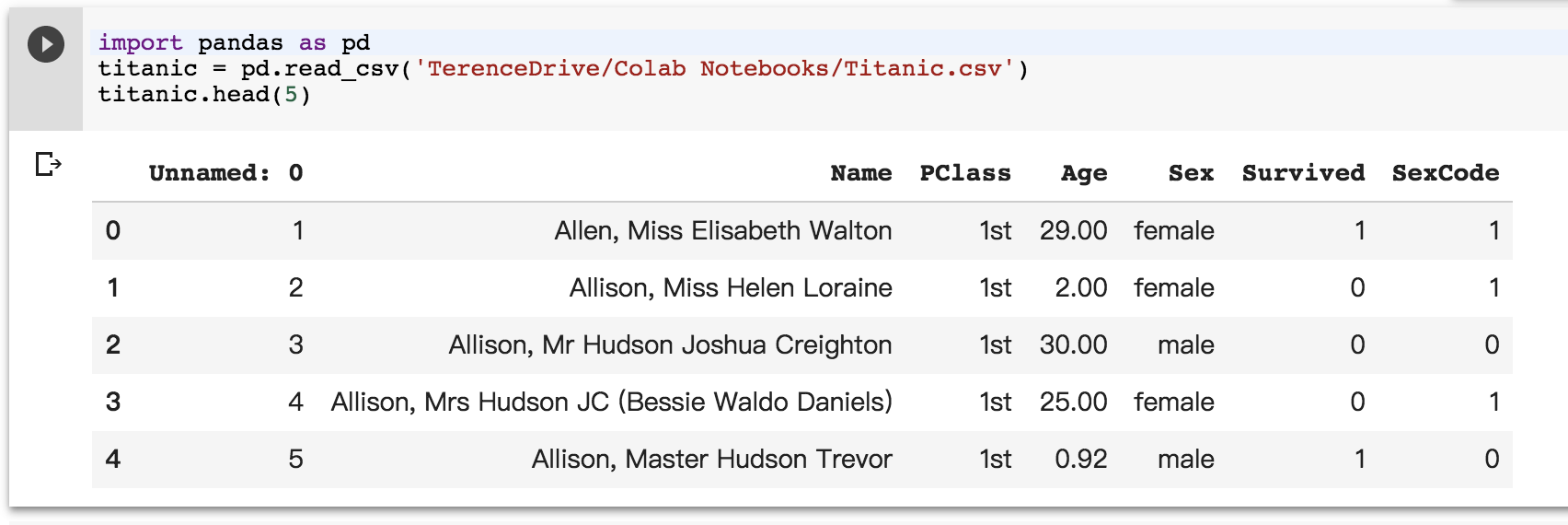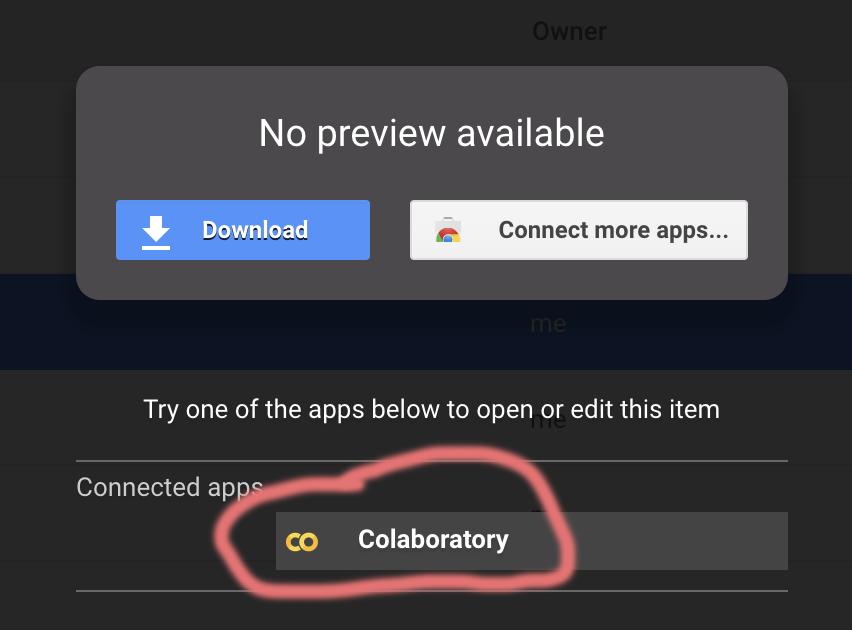## 为什么要使用Colab## 如何使用Colab### 如何使用GPU### 安装程序库

 !pip install -q keras

!pip install -q matplotlib-venn

# To determine which version you're using:
!pip show tensorflow
# For the current version:
# For a specific version:
!pip install tensorflow==1.2
# For the latest nightly build:
!pip install tf-nightly

# Check your current directory
!pwd
# Check contents under current directory
!ls

!apt-get install -y -qq software-properties-common python-software-properties module-init-tools
!apt-get update -qq 2>&1 > /dev/null
!apt-get -y install -qq google-drive-ocamlfuse fuse
auth.authenticate_user()
import getpass
vcode = getpass.getpass()
!echo {vcode} | google-drive-ocamlfuse -headless -id={creds.client_id} -secret={creds.client_secret}!mkdir -p TerenceDrive
!google-drive-ocamlfuse TerenceDrive

### Colab中运行.py文件

'''This is [mnist_cnn.py]'''

'''Trains a simple convnet on the MNIST dataset.
Gets to 99.25% test accuracy after 12 epochs
(there is still a lot of margin for parameter tuning).
16 seconds per epoch on a GRID K520 GPU.
'''
from __future__ import print_function
import keras
from keras.datasets import mnist
from keras.models import Sequential
from keras.layers import Dense, Dropout, Flatten
from keras.layers import Conv2D, MaxPooling2D
from keras import backend as K
batch_size = 128
num_classes = 10
epochs = 12
# input image dimensions
img_rows, img_cols = 28, 28
# the data, split between train and test sets
(x_train, y_train), (x_test, y_test) = mnist.load_data()
if K.image_data_format() == 'channels_first':
x_train = x_train.reshape(x_train.shape, 1, img_rows, img_cols)
x_test = x_test.reshape(x_test.shape, 1, img_rows, img_cols)
input_shape = (1, img_rows, img_cols)
else:
x_train = x_train.reshape(x_train.shape, img_rows, img_cols, 1)
x_test = x_test.reshape(x_test.shape, img_rows, img_cols, 1)
input_shape = (img_rows, img_cols, 1)
x_train = x_train.astype('float32')
x_test = x_test.astype('float32')
x_train /= 255
x_test /= 255
print('x_train shape:', x_train.shape)
print(x_train.shape, 'train samples')
print(x_test.shape, 'test samples')
# convert class vectors to binary class matrices
y_train = keras.utils.to_categorical(y_train, num_classes)
y_test = keras.utils.to_categorical(y_test, num_classes)
model = Sequential()
activation='relu',
input_shape=input_shape))
model.compile(loss=keras.losses.categorical_crossentropy,
metrics=['accuracy'])
model.fit(x_train, y_train,
batch_size=batch_size,
epochs=epochs,
verbose=1,
validation_data=(x_test, y_test))
score = model.evaluate(x_test, y_test, verbose=0)
print('Test loss:', score)
print('Test accuracy:', score)

!python3 'TerenceDrive/Colab Notebooks/mnist_cnn.py'

!wget https://raw.githubusercontent.com/vincentarelbundock/Rdatasets/master/csv/datasets/Titanic.csv -P 'TerenceDrive/Colab Notebooks'

import pandas as pd
titanic.head(5)### Colab中使用GitHub Repo

!git clone https://github.com/wxs/keras-mnist-tutorial.git 'TerenceDrive/Colab Notebooks/keras-mnist-tutorial'VIP赞赏专区posted @ 2018-03-11 17:38  kid551  阅读(...)  评论(...编辑  收藏